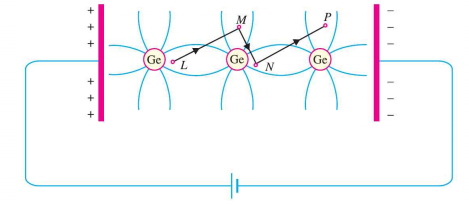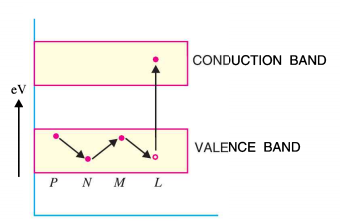# Hole Current In Semiconductor

## Hole Current In Semiconductor

At room temperature, some of the co-valent bonds in a pure semiconductor crystal break, thus setting up free electrons. Under the influence of  electric field, these free electrons constitute electric current.

At the same time, another current i.e. hole current, also flows in the semiconductor.

When a co-valent bond is broken due to thermal energy, the removal of one electron leaves a vacancy i.e. a missing electron in the co-valent bond. This missing electron is called a hole which acts as a positive charge.

For one electron set free, one hole is created. Therefore, thermal energy creates hole-electron pairs. That means number of  free electrons is equal to number of holes.

The current conduction by holes can be explained as follows:Fig.1

Let us consider the valence electron at L .

Due to thermal energy this valence electron will become a free electron.

This will create a hole in the co-valent bond at L.

Since, hole is a strong centre of attraction for the electrons, a valence electron  at M, from nearby covalent-bond will come to fill in the hole at L.

This will result in the creation of hole at M.

Another valence electron at N in turn will leaves its co-valent bond to fill the hole at M, thus creating a hole at N.

Thus the hole having a positive charge will move from L to N i.e. towards the negative terminal of supply.

This will  constitute hole current.

### Energy Band Description

The hole current can be explained easily in terms of energy bands.

Let us consider an electron in valence band.

Due to thermal energy this valence electron will leave the valence band and enter into the conduction band as shown in the Fig.2 below.Fig.2

This will leave a vacancy at L , thus creating a hole there.

Now the valance electron at M will come to fill the hole at L.

This will result that the hole will disappear at L and appear at M.

Consequently, hole will be created at N.

It can be noted from the fig. that valence electrons move along the path PNML whereas, holes move  along the path LMNP.